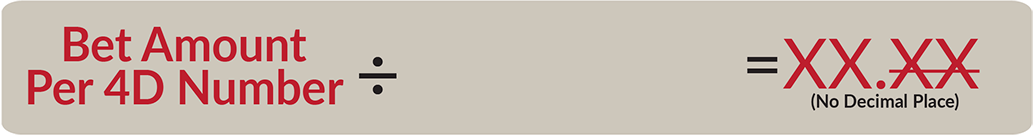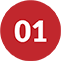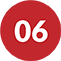## QUALIFIED BONUS WINNER

All Bonus Jackpot is only applicable our 4D BIG, and 4D SMALL product. The minimum bet per 4D number must not less than USD1 (or equal to RM4 / THB30 / IDR14,000), and it's not including the combination of USD1 (or equal to USD1 / RM4 / THB30 / IDR14,000) for both SMALL and BIG 4D. E.g. If any user who purchased 1234 with RM2 SMALL and RM2 BIG. This combination of RM4 is not qualified for one unit of Bonus Jackpot (as per 4D number is only RM2). You will earn 1 unit share of the Bonus for every USD1 bet for each individual 4D number, and earn 2 units of share if your bet exceed USD1.99.
 COUNTRY QUALIFIED UNIT PRICE Cambodia USD1 - USD1.99 1 unit Malaysia MYR4 - MYR7.99 1 unit Thailand THB30 - THB59.9 1 unit Indonesia IDR14,000 - IDR27,999 1 unit

## FORMULA OF A QUALIFIED BONUS UNIT

How to count a unit of qualified Bonus Unit?USD 1.00

## FOR EXAMPLE:Betting of USD1 per 4D number.
\$1 / \$1 = 1 unitBetting of USD2 per 4D number.
\$2 / \$1 = 2 unitsBetting of USD3.50 per 4D number.
\$1.50 / \$1 = 1 unit (1.5 = 1)Betting of USD4.5 per 4D number.
\$4.50 / \$1 = 4 units (4.50 = 4)Betting of USD7 per 4D number.
\$7 / \$1 = 7 unitsBetting of USD7.25 per 4D number.
\$7.25 / \$1 = 7 units (7.25 = 7)

\$   /  \$1
Calculate

# 0 units

\$   /  \$1
Calculate

## 0 units

How to count a unit of qualified Bonus Unit?RMB 7.00

## FOR EXAMPLE:Betting of ¥7 per 4D number.
¥7 / ¥7 = 1 unitBetting of ¥14 per 4D number.
¥14 / ¥7 = 2 unitsBetting of ¥10.50 per 4D number.
¥10.50 / ¥7 = 1 unit (1.5 = 1)Betting of ¥31.5 per 4D number.
¥31.5 / ¥7 = 4 units (4.50 = 4)Betting of ¥49 per 4D number.
¥49 / ¥7 = 7 unitsBetting of ¥50.75 per 4D number.
¥50.75 / ¥7 = 7 units (7.25 = 7)

¥   /  ¥7
Calculate

# 0 units

¥   /  ¥7
Calculate

## 0 units

How to count a unit of qualified Bonus Unit?RM 4.00

## FOR EXAMPLE:Betting of RM4 per 4D number.
RM4 / RM4 = 1 unitBetting of RM8 per 4D number.
RM8 / RM4 = 2 unitsBetting of RM7.50 per 4D number.
RM7.50 / RM4 = 1 unit (1.875 = 1)Betting of RM18 per 4D number.
RM18 / RM4 = 4 units (4.50 = 4)Betting of RM28 per 4D number.
RM28 / RM4 = 7 unitsBetting of RM29 per 4D number.
RM29 / RM4 = 7 units (7.25 = 7)

RM   /  RM4
Calculate

# 0 units

RM   /  RM4
Calculate

## 0 units

How to count a unit of qualified Bonus Unit?IDR 14,000

## FOR EXAMPLE:Betting of IDR14,000 per 4D number.
IDR14,000 / IDR14,000 = 1 unitBetting of IDR24,000 per 4D number.
IDR28,000 / IDR14,000 = 2 unitsBetting of IDR18,000 per 4D number.
IDR21,000 / IDR14,000 = 1 unit (1.5 = 1)Betting of IDR54,000 per 4D number.
IDR63,000 / IDR14,000 = 4 units (4.50 = 4)Betting of IDR84,000 per 4D number.
IDR98,000 / IDR14,000 = 7 unitsBetting of IDR90,000 per 4D number.
IDR101,500 / IDR14,000 = 7 units (7.25 = 7)

### Qualified Bonus Unit Calculator

IDR   /  IDR14,000
Calculate

# 0 units

#### Qualified Bonus Unit Calculator

IDR   /  IDR14,000
Calculate

## 0 units

How to count a unit of qualified Bonus Unit?30 BAHT

## FOR EXAMPLE:Betting of ฿30 per 4D number.
฿30 / ฿30 = 1 unitBetting of ฿60 per 4D number.
฿60 / ฿30 = 2 unitsBetting of ฿56.25 per 4D number.
฿56.25 / ฿30 = 1 unit (1.875 = 1)Betting of ฿120 per 4D number.
฿120 / ฿30 = 4 units (4.50 = 4)Betting of ฿210 per 4D number.
฿210 / ฿30 = 7 unitsBetting of ฿217.50 per 4D number.
฿217.50 / ฿30 = 7 units (7.25 = 7)

฿   /  ฿30
Calculate

# 0 units

฿   /  ฿30
Calculate

## BONUS JACKPOT SYSTEM